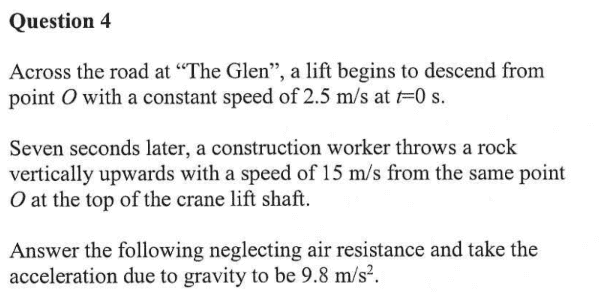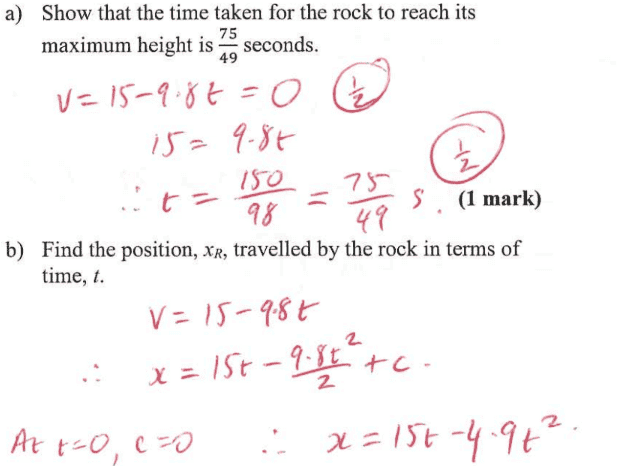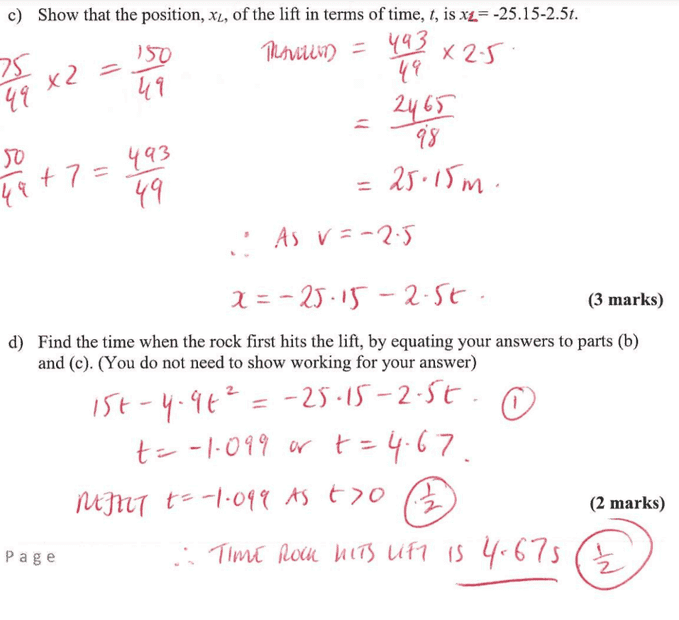# Questioning the Solution for Elevator vs Rock Problem

• Darkmisc
In summary, the author of the problem is concerned about when the rock returns to the elevator's starting point. They say that the rock has a velocity of -15m/s when it returns to the origin and that the lift is at -2.5m/s from the origin. They also say that the clock is started when the rock is thrown and that the lift is at its position when the rock is thrown. They propose that the lift moves for about 10s so that the elevator can get to -25.15m and the rock can reach its peak and return to X =0. They then reset the clock to t=0.f

#### Darkmisc

Homework Statement
An elevator descends downwards at 2.5m/s. Seven seconds after this, a rock is thrown upwards at 15m/s. g=9.8m/s^2. When will the rock hit the elevator?
Relevant Equations
v = 15 -9.8t.
Hi everyone

I have solutions to this problem, but I am not sure if the premise behind them is correct.

I think this is the reasoning behind the solution for question d.

- Work out how far the elevator travels in the 7s before the rock is thrown, so 7x2.5 = 17.5
- Work out how far the elevator travels in the time it takes for the rock to reach its peak and then fall back to X=0, so 150/49 x 2.5 = 7.65
- This means the elevator will have a head start of 25.15m on the rock when the rock returns to X = 0.
- After this point, the position of the elevator can be given by XL = -25.15 -2.5t

The part of the solution that I'm not sure about is where they give the position of the rock with XR= 15t - 4.9t^2.

It seems like they are starting the clock with XL at -25.15 and XR at 0. However, at this point in time, XR is not starting from rest. It had already fallen for 75/49s. I'm not sure that the equation XR=15t - 4.9t^2 accounts for this.

When the rock returns to X =0, its velocity should be 15 - 150/49*9.8 = -15m/s. Its velocity at subsequent moments should be given by v=-15 -9.8t. Integrating this should give XR= -15t -4.9t^2. I think it is this equation for XR that should be equated with -25.15 -2.5t to get the correct value for t.

Is my reasoning correct? Or are the solutions correct?

Thanks

https://www.physicsforums.com/attachments/313668

https://www.physicsforums.com/attachments/313669

https://www.physicsforums.com/attachments/313671

I can't view the attachments and I can't follow what you are saying. The best idea is to start the clock when the rock is thrown up.

•topsquark
I can't view the attachments either.

Is the rock above or below the elevator when it is projected upwards? How far is it from the elevator when the elevator starts moving? That information is important for finding when the rock hits the elevator.

•topsquark
Sorry, not sure what went wrong with the attachments•topsquark
Parts (a) and (b) look fine.

I disagree with the author of the problem about part (c). Here is why.
Both rock and lift start at point O. This is the origin from which positions are measured.
Time ##t## is measured with respect to a clock that starts when the rock is thrown.
At that time, the lift is at position ##D=-2.5~\text{(m/s)}\times 7~\text{s}=-17.5~\text{m}## relative to the origin. I don't see where the ##x_L(t)=-25.15-2.5t## is coming from. It predicts that at time ##t=0## the lift is at ##-25.15## m which would be its position if it started moving about 10 s before the rock. Am I missing something?

Part (d) is consistent with part (c).

•Darkmisc, PeroK and topsquark
Well, it says in the question that ##t = 0## when the lift starts to move and the rock is thrown at ##t = 7s##. That doesn't seem to be respected in the solution.

•Darkmisc and SammyS
Parts (a) and (b) look fine.

I disagree with the author of the problem about part (c). Here is why.
Both rock and lift start at point O. This is the origin from which positions are measured.
Time ##t## is measured with respect to a clock that starts when the rock is thrown.
At that time, the lift is at position ##D=-2.5~\text{(m/s)}\times 7~\text{s}=-17.5~\text{m}## relative to the origin. I don't see where the ##x_L(t)=-25.15-2.5t## is coming from. It predicts that at time ##t=0## the lift is at ##-25.15## m which would be its position if it started moving about 10 s before the rock. Am I missing something?

Part (d) is consistent with part (c).
I think the solution let's the clock run for about 10s so that the elevator can get to -25.15m and the rock can reach its peak and return to X =0. It then resets the clock to t=0. I think this was the author's way of dealing with the 7s difference between the elevator's descent and the rock being thrown.

I also don't agree with the author's approach. It confused me and I think they've jumped between time frames without making it clear that's what they did. The answer they gave is 4.67s, which is less than the time it took for the elevator to get to -25.15m. I'm guessing they mean to add 4.67s to the ~10s, but even then, I think it'll be the wrong answer.

I think the solution let's the clock run for about 10s so that the elevator can get to -25.15m and the rock can reach its peak and return to X =0.
You can't reverse-engineer the problem statement from the solution and try to justify the answer this way. The problem statement should be written in such a way that the solution can be found without ambiguity. It seems to me that the author of the problem changed the input numbers and forgot to change the equation in part (c) to match the new numbers.

For future reference, the way to handle problems is to write separate equations for each moving object in which time starts when the object starts moving and use subscripts to keep the objects sorted out. For the lift, the equation is $$x_L=V_Lt_L$$ For the rock it is $$x_R=v_0t_R-\frac{1}{2}gt_R^2.$$Now the rock clock is ##t_0=7## s behind the lift clock. In other words, ##t_R=t_L-t_0## which makes the rock equation $$x_R=v_0t_L-\frac{1}{2}g(t_L-t_0)^2.$$ At specific time ##t_L=T## the lift and the rock are at the same place, i.e. the rock collides with the lift. This means that $$v_0T-\frac{1}{2}g(T-t_0)^2=V_LT.$$ At this point you solve the quadratic in ##T## with ##v_0=15## m/s, ##V_L=-2.5## m/s, ##t_0=7## s and ##g=9.8## m/s2.

•PeroK and Darkmisc